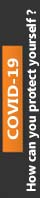2019-01-25T10:11:36+00:00 Assignments

# Correlation of spot exchange rate

This paper concentrates on the primary theme of Correlation of spot exchange rate in which you have to explain and evaluate its intricate aspects in detail. In addition to this, this paper has been reviewed and purchased by most of the students hence; it has been rated 4.8 points on the scale of 5 points. Besides, the price of this paper starts from £ 40. For more details and full access to the paper, please refer to the site.

# Correlation of spot exchange rate

Correlation Case One

 In the following calculations, we all use data after taking natural logarithm except for interest rates. (a) Correlation of spot exchange rate and Aust. MS is: – (for example, this uses data of “log of S(AUD/NZD)” and “log of Aust. MS”, all the following means the same way) 0.461763 Correlation of spot exchange rate and NZ MS is: 0.529167 Correlation of spot exchange rate and Aust. GDP is: 0.495649 Correlation of spot exchange rate and NZ GDP is: 0.509683 Correlation of spot exchange rate and Aust. IR is: -0.48547 Correlation of spot exchange rate and NZ IR is: -0.44792 So we could see the strongest correlation is the one of spot exchange rate and NZ MS, which is 0.529167, a positive correlation. So NZ MS is our “PREDICTOR”.

(b)

 Mean of log of spot exchange rate: -0.19909 Median of log of spot exchange rate: -0.20046 Mode of log of spot exchange rate: #N/A

Mean is the arithmetic average of the data; and median is the number which positions in the middle of the data, which means 50% of the data are below median and another 50% of the data are higher than the median; and mode refers to the number which appears most frequently in the data. And there is no mode in this data set.

The value of mean is -0.19909, which is just a little higher than the median which is -0.20046. I think mean is the most appropriate measure of average here because there isn’t any extreme value in the data set.

(c)

The minimum of the log of spot exchange rate is -0.55644, and the maximum of the log of spot exchange rate is -0.04051, so I chose the range -0.56 to -0.01 to draw the histogram.

Histogram of log of spot exchange rate:

 Variance: 0.008038205 Skewness: -0.99690259 Kurtosis: 2.28480724

As calculated in question (b), the mean is close but not equal to median, so this data is not symmetric. And this is further demonstrated by the value of skewness, which is -0.9969, and which is not equal to 0. This is because if the data is symmetric, its skewness should be equal to 0.

If the data follows a normal distribution, the value of its kurtosis must be equal to 3. But now the value of kurtosis is 2.2848, which indicates the data does not follow a normal distribution.

So based on the above results, the log of spot exchange rate does not have a normal distribution.

(d)

• The level of the New Zealand money supply is our “PREDICTOR” as it has the strongest correlation with spot exchange rate.

• And mean is the most appropriate average for the log of spot exchange rate.

• Also the log of spot exchange rate does not have a normal distribution.

0% Plagiarism Guaranteed & Custom Written, Tailored to your instructions

International House, 12 Constance Street, London, United Kingdom,
E16 2DQ

## STILL NOT CONVINCED?

We've produced some samples of what you can expect from our Academic Writing Service - these are created by our writers to show you the kind of high-quality work you'll receive. Take a look for yourself!FLAT 25% OFF ON EVERY ORDER.Use "FLAT25" as your promo code during checkout